• 多重分形谱，用于时间序列分析，是多重分形分析重要的分析环节。
• 根据 Posadas 等人在 Soil Sci 的论文中的思想计算二值图像的多重分形谱。 社会。 是。 J. 67:1361-1369, 2003。matlab
• 多重分形谱计算程序matlab，可用于股市分析等。有问题希望大家及时反馈给我。 多重分形谱计算程序matlab，可用于股市分析等。有问题希望大家及时反馈给我。
• 该程序应用于一维数据的多重分形分析，是matlab代码
• 针对单一分形维数在高光谱数据处理中的不足,提出了一种基于多重分形谱的光谱信号奇异性特征提取方法,引入多重分形谱表征光谱曲线的奇异性特征。该方法根据分形测度将光谱曲线进行划分,用光谱概率测度计算配分函数,...
• ## WTMM多重分形谱

千次阅读 2020-10-07 20:41:38
通过多重分形谱仿真，得到如下的仿真结果： 然后进行分类，得到如下的仿真结果： 神经网络参数如下所示： 前面得到的特征数据集如下所示： 得到的分类结果如下所示： 上面蓝色的表示两类...
一、仿真结果分析
所利用的测试数据为：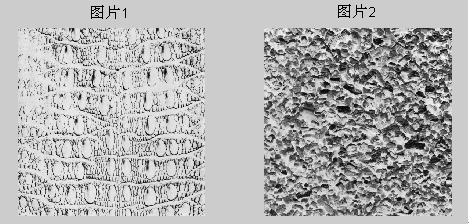通过多重分形谱仿真，得到如下的仿真结果：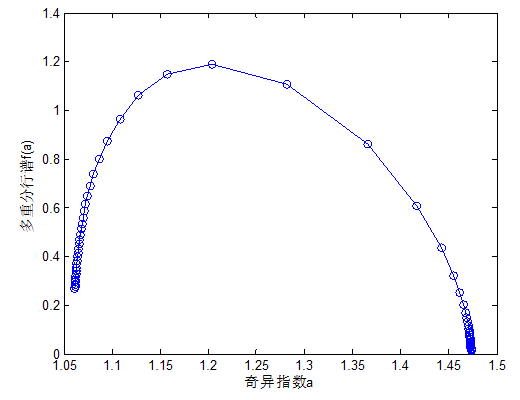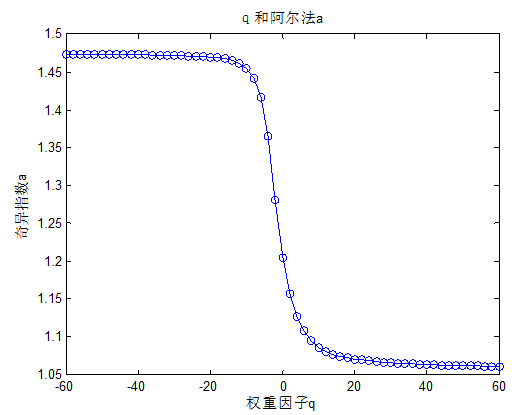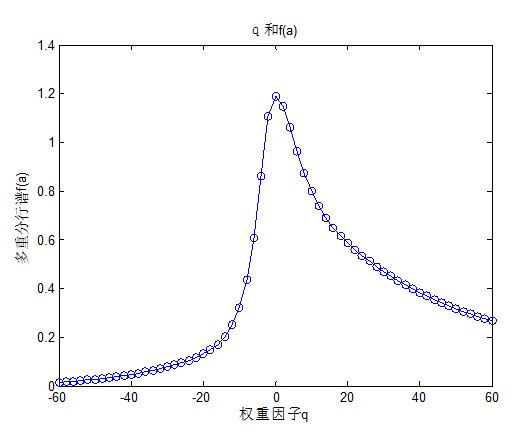然后进行分类，得到如下的仿真结果：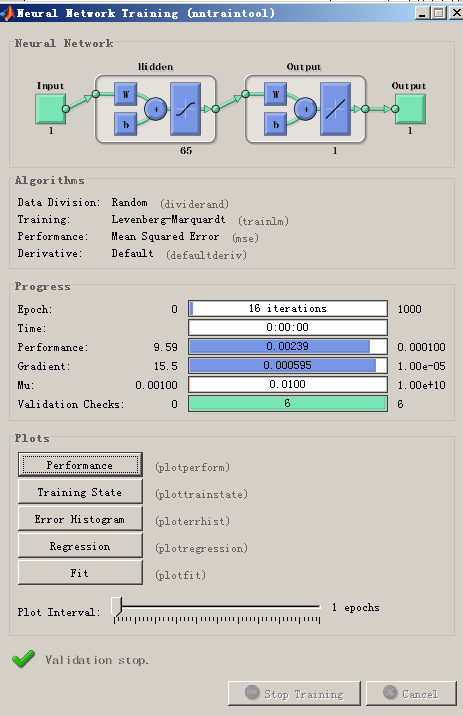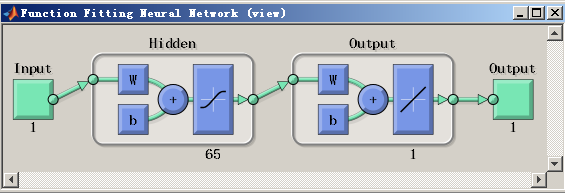神经网络参数如下所示：
前面得到的特征数据集如下所示：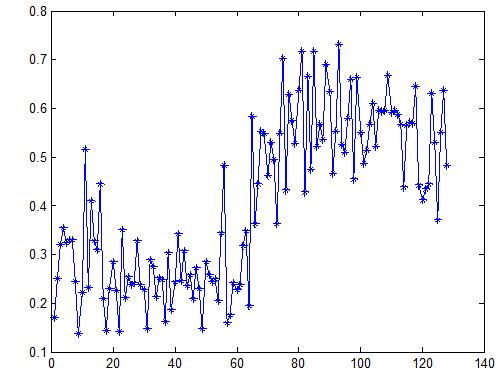得到的分类结果如下所示：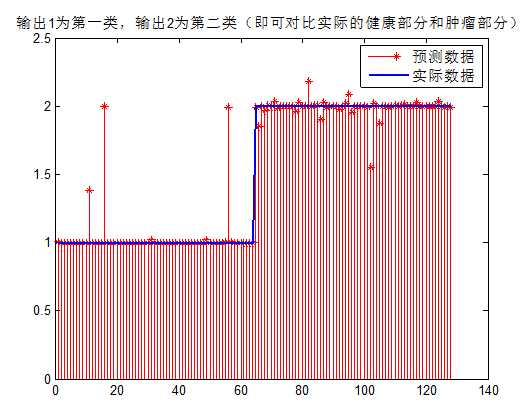上面蓝色的表示两类图片，1表示第一个图片，2表示第二个图片。
红色部分表示对64个子图进行分类，通过统计计算，可以得到的分类的正确率达到了：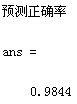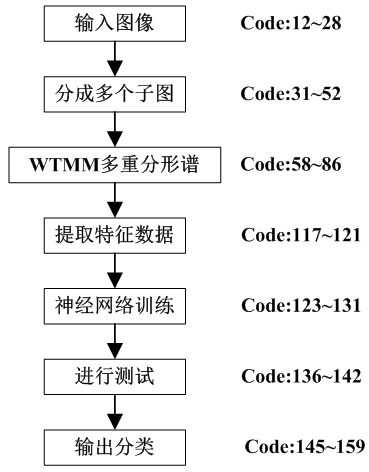三、代码使用说明
首先设置sel = 1：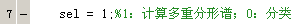，进行仿真。计算多重分形谱，然后提取特征参数。
然后设置sel = 0；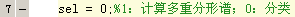，进行仿真。利用神经网络进行分类，得到最后的子图片分类结果。
展开全文• 研究结果显示 ,多重分形谱宽度的变化与网络流量的变化十分相似 ,且最大与最小概率的多重分形谱函数之差的变化在某种程度上比流量的波动要提前一个时段 ,该结果为采用多重分形谱实现网络流量预测奠定了理论基础。
•研究论文
• 针对湿式离合器试验过程中磨合状态的预测问题，结合试验过程中摩擦转矩信号的几何结构特征，提出利用多重分形谱及其参数来预测磨合状态的新方法。阐述了基于盒计数法计算多重分形谱的方法；给出了多重分形谱参数的...
• matlab开发-二值图像的多重分形谱。输入二进制图像。程序计算图像的多重分形光谱。
•研究论文
• 可以求出二值图片的多重分形谱，从而分析研究对象的分布均匀性，，当然也可以很方便地延伸至三维的云图分析。。
• 系统阐述了多重分形的概念和多重分形谱的小波模极大值计算方法,提出了一种新的基于动态电流IDDT多重分 形谱分析的集成电路故障诊断方法。该方法利用多重分形分析方法有效地提取信号的几何结构特征信息,利用动态电流...
• 给出了齐次自相似集满足分离性质的一个充分条件，对于直线上的一致压缩映射族，若齐次压缩比小于各个压缩映射平移量之间的最小距离，则相应齐次自相似集满足开集条件，从而它的各种多重分形谱都相等，于是得到了重...
• 输入灰度图像,RGB彩色图像会自动转换为灰度图像 输出图像的多重分形谱 参考文献： "fractal analysis and multifractal spectra for the images
• 将多重分形理论引入到植物叶片的图像处理中，用数字图像处理与分析技术，重点对葫芦科的4种植物叶片的图像进行处理，通过计算多重分形谱分析植物叶片的特点。该文处理叶片图像的方法，可望在植物分类及农业生产中...
•matab
• 着重讨论了基于P2P结构的移动对象kNN组查询算法。组查询算法采用建立计时区间的方法合并计时器，并通过改变临界物体的应答模式来优化临界物体间的通信协议。为降低移动对象资源的紧张程度，设计了区间计时算法和临界...
• 正因为这种假设的不合理性，使得分类效果和识别性能有限，引入以数据相关性为核心的多重分形理论，从根本上摈弃独立假设的局限性与狭隘性，实现流的有效分类。为此，定义并论证流的分形谱，在此基础上推导流的估计谱...数据相关性 灰色关联度
• 采用 多重分形谱纹理分析法 以及模式识 别技术 ， 建立一 种识别正 常肝脏 和不 同程度脂 肪肝 B超 图像 的 计算 机辅 助诊 断方法 。方法 通过提取每幅 B超 图像 多重分 形谱 曲线 的奇异标 度差 和多重谱 面积两 ...
• % figure(1) % plot(X',Y,'o-k') % 至此，计算多重分形谱的第一步，分配函数构建完毕 side_length= tmin:1:tmax; % 自定义网格边长，“※—5” side_length=side_length'; q=ttmin:ttmax; % q=-5:1:n-10，q=-10:...

function multifractal(A)
format long g
L=length(A);
i=1;
modify=1;
tmin=2;                % 边框间距，“※”
tmax=10;
ttmin=-10;
ttmax=10;               % 自定义 q 的范围
for r=tmin:1:tmax
c(i,1)=mod(L,r);
i=i+1;
end
c';                     % 计算不能被边长r整除的余数
a=L-c';                 % 计算并剔除掉不能被边长r整除的原始数据
n=length(a);            % 求解格网化边长的个数，即为 n
TT=[];
j=1;
r=tmin;                % 自定义项，“※-2”
for i=1:1:n             % 即n=25-10+1，自定义的结果
B=A(1:a(i),1);
U=reshape(B,r,length(B)/r);
T=mean(U);
T=T'.*r^3;
TT(1: length(T),i)=[T];
modifying(modify,1)=length(T);
r=r+1;
if r>= tmin+n           % 自定义项，“※-3”
break;             % 边长超过10+n，超过初始限制，则程序自动终止
end
modify=modify+1;
end
modifying;
TT= nthroot(TT,1);
% 或者不缩小
% TT;
TT=nonzeros(TT);
for cugb=1:1:n
modifying_modifying(cugb,1)=sum(modifying(1:cugb));     % 有影响的新加卷 % & * % ￥ # @ ！) ……
end
modifying_modifying;
j=1;
% q 为任意数，这里取1到n，为n，与 k取值保持一致，q过大，计算机无法
%识别，默认为无穷大，q过小，结果接近0，则意义不明确
for q=ttmin:ttmax           %这里取 q=-10:1:10
for k=1:1:n
X=TT(1:modifying_modifying(k,1),1).^q;
if k>1
X=TT(modifying_modifying(k-1,1)+1:modifying_modifying(k,1),1).^q;
end
t=sum(X);
XX(k,j)=[t];             % 这里用到两个循环，即考虑到了幂函数，又需考虑求和
end
j=j+1;
end
XX;                     % 得到质量分配函数，Xq(ξ)，
X=log(tmin:1:tmax);
% X=log(tmax:-1:tmin);          % 此系以前的自定义输入结果，“※—4”
Y=log(XX);
% figure(1)
% plot(X',Y,'o-k')        % 至此，计算多重分形谱的第一步，分配函数构建完毕
side_length= tmin:1:tmax;         % 自定义网格边长，“※—5”
side_length=side_length';
q=ttmin:ttmax;                    % q=-5:1:n-10，q=-10:1:10
m=1;
[ha,hb]=size(XX);
for i=1:1:hb
% XX=XX’;                         % or not
s=XX(:,i);                        % XX
b=polyfit(log(side_length),log(s),1);  % 在对数尺度下计算斜率
slope(m,1)=b(1,1);
m=m+1;
end
slope;                                 %  这里的Slope即为质量指数，τ(q)
N=polyfit(q', slope,1);
plot(q', slope)    %  此步是考察τ(q)-q 之间的关系，
a=diff(slope)./diff(q');   % 第三步计算，diff函数求偏导确实少一列
q=q';
f_a=a.*q(1:end-1)-slope(1:end-1);
% figure(2)
% a=sort(a,'ascend');
plot(a,f_a,'o-k')
xlabel('α','FontSize',12);
ylabel('f(α)','FontSize',12);
% polyfit(a,f_a,3)
a=sort(a,'ascend');
% a+1
% f_a+1
a    % 奇异性指数
f_a % 不同层次的分维数

展开全文• 为深入分析煤样受载破裂机制,试验研究了煤样单轴压缩下表面电位变化规律,应用多重分形统计理论分析表面电位信号,计算其多重分形谱,并分析多重分形谱特征参数随加载过程的变化规律。研究结果表明:煤样表面电位信号...
• 此外，H(q) 和 H(t) 还用于通过 H(q) 的勒让德变换或直接从 H(t) 的直方图计算多重分形谱 D(h)。 如果这些代码用于科学出版物，请引用 zip 文件夹中包含的 Ihlen (2012)。 小波和EMD趋势化的MFDFA代码的修改在菱...matlab
• 采用多重分形理论、物理试验相结合的方法研究了试件单轴压缩破坏过程中电磁辐射信号的多重分形谱宽度ΔD_q的变化规律。研究结果表明:试件单轴压缩破坏过程中产生的电磁辐射信号具有多重分形特征;多重分形谱宽度ΔD_q...
• 深入研究了多重分形维数Dq和多重分形谱函数f（aq）随矩q的变化。 发现Dq随着瞬间量级q的增加而减小，从而表明在多颗粒生产中对于考虑的碰撞具有自相似行为。 我们还发现了多重分形谱函数的向下凹曲线，其最大q = 0......